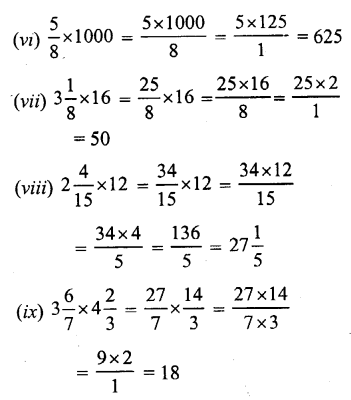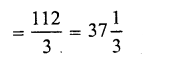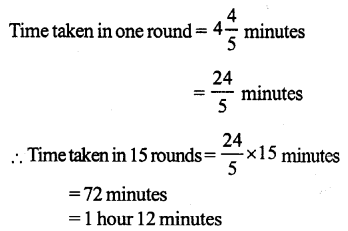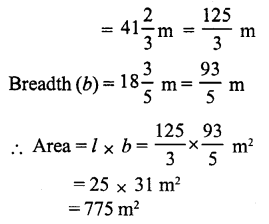# RS Aggarwal Solutions Class 7 Chapter 2 Fractions Ex 2B

In this chapter, we provide RS Aggarwal Solutions for Class 7 Chapter 2 Fractions Ex 2B for English medium students, Which will very helpful for every student in their exams. Students can download the latest RS Aggarwal Solutions for Class 7 Chapter 2 Fractions Ex 2B Maths pdf, free RS Aggarwal Solutions Class 7 Chapter 2 Fractions Ex 2B Maths book pdf download. Now you will get step by step solution to each question.

### RS Aggarwal Solutions Class 7 Chapter 2 Fractions Ex 2B Download PDF

Question 1.
Solution:Question 2.
Solution:Question 3.
Solution:Question 4.
Solution:Question 5.
Solution:
Cost of 1 metre pf clothQuestion 6.
Solution:
Distance covered in 1 hourQuestion 7.
Solution:Question 8.
Solution:Question 9.
Solution:Question 10.
Solution:Question 11.
Solution:
Weight of Amit = 35 kg.Question 12.
Solution:
Total number of students in a class = 42
Number of boys = 57 of 42 = 5 x 6 = 30
Number of girls = 42 – 30 = 12

Question 13.
Solution:
Sapna total income for one month = Rs 24000
Amount spent = 78 of her income
= 78 x 24000
= Rs (7 x 3000) = Rs 21000
Amount deposited in the bank per month = Rs 24000 – 21000 = Rs 3000

Question 14.
Solution:
Length of each side of a squareQuestion 15.
Solution:
Length of rectangular field (l)All Chapter RS Aggarwal Solutions For Class 7 Maths

—————————————————————————–

All Subject NCERT Exemplar Problems Solutions For Class 7

All Subject NCERT Solutions For Class 7

*************************************************

I think you got complete solutions for this chapter. If You have any queries regarding this chapter, please comment on the below section our subject teacher will answer you. We tried our best to give complete solutions so you got good marks in your exam.

If these solutions have helped you, you can also share rsaggarwalsolutions.in to your friends.